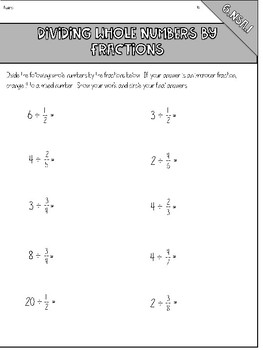# Dividing Whole Number by Fractions Notes & Practice Pages6th
Subjects
Standards
Resource Type
Formats Included
• PDF
Pages
7 pages

### Description

6.NSA.1

Included are three practice pages for practicing dividing whole numbers by fractions.

Includes:

-Notes page with 6 practice problems

-Additional practice page with 10 practice problems (use for a quiz, quick check, homework, etc!)

-Word problem practice page with 5 practice word problems

Total Pages
7 pages
Included
Teaching Duration
N/A
Report this Resource to TpT
Reported resources will be reviewed by our team. Report this resource to let us know if this resource violates TpT’s content guidelines.

### Standards

to see state-specific standards (only available in the US).
Interpret and compute quotients of fractions, and solve word problems involving division of fractions by fractions, e.g., by using visual fraction models and equations to represent the problem. For example, create a story context for (2/3) ÷ (3/4) and use a visual fraction model to show the quotient; use the relationship between multiplication and division to explain that (2/3) ÷ (3/4) = 8/9 because 3/4 of 8/9 is 2/3. (In general, (𝘢/𝘣) ÷ (𝘤/𝘥) = 𝘢𝘥/𝘣𝘤.) How much chocolate will each person get if 3 people share 1/2 lb of chocolate equally? How many 3/4-cup servings are in 2/3 of a cup of yogurt? How wide is a rectangular strip of land with length 3/4 mi and area 1/2 square mi?